This document provides a few examples of Analyses of Variance for typical experimental designs. We recommend to use of the `ez` package to perform ANOVAs with R.

``require(ez)``
``## Loading required package: ez``
``require(lattice)``
``## Loading required package: lattice``
``require(ggplot2)``
``## Loading required package: ggplot2``

# S<G> Design (one-way ANOVA between subjects)

We start by analyzing a design where the factor Subject is nested within the factor Group, that is, we want to compare independent groups.

``````ns <- c(20, 30, 20, 15)
means <- c(100, 110, 100, 130)
sd <- c(20, 20, 30, 25)

dat1 <- data.frame(
subj=factor(1:sum(ns)),
group=factor(rep(1:4, ns)),
y=c(rnorm(ns, means, sd),
rnorm(ns, means, sd),
rnorm(ns, means, sd),
rnorm(ns, means, sd)))``````

Let us inspect the data:

``str(dat1)``
``````## 'data.frame':    85 obs. of  3 variables:
##  \$ subj : Factor w/ 85 levels "1","2","3","4",..: 1 2 3 4 5 6 7 8 9 10 ...
##  \$ group: Factor w/ 4 levels "1","2","3","4": 1 1 1 1 1 1 1 1 1 1 ...
##  \$ y    : num  75.9 108.9 148.1 80.3 63.4 ...``````
``head(dat1)``
``````##   subj group         y
## 1    1     1  75.85807
## 2    2     1 108.91287
## 3    3     1 148.09366
## 4    4     1  80.28648
## 5    5     1  63.41147
## 6    6     1 111.60601``````

## Visual inspection

Different graphics can be used, the best choiice depends on the number of data points. For example, dotplots are good when there are not too many points:

``````require(lattice)
with(dat1, dotplot(y ~ group))``````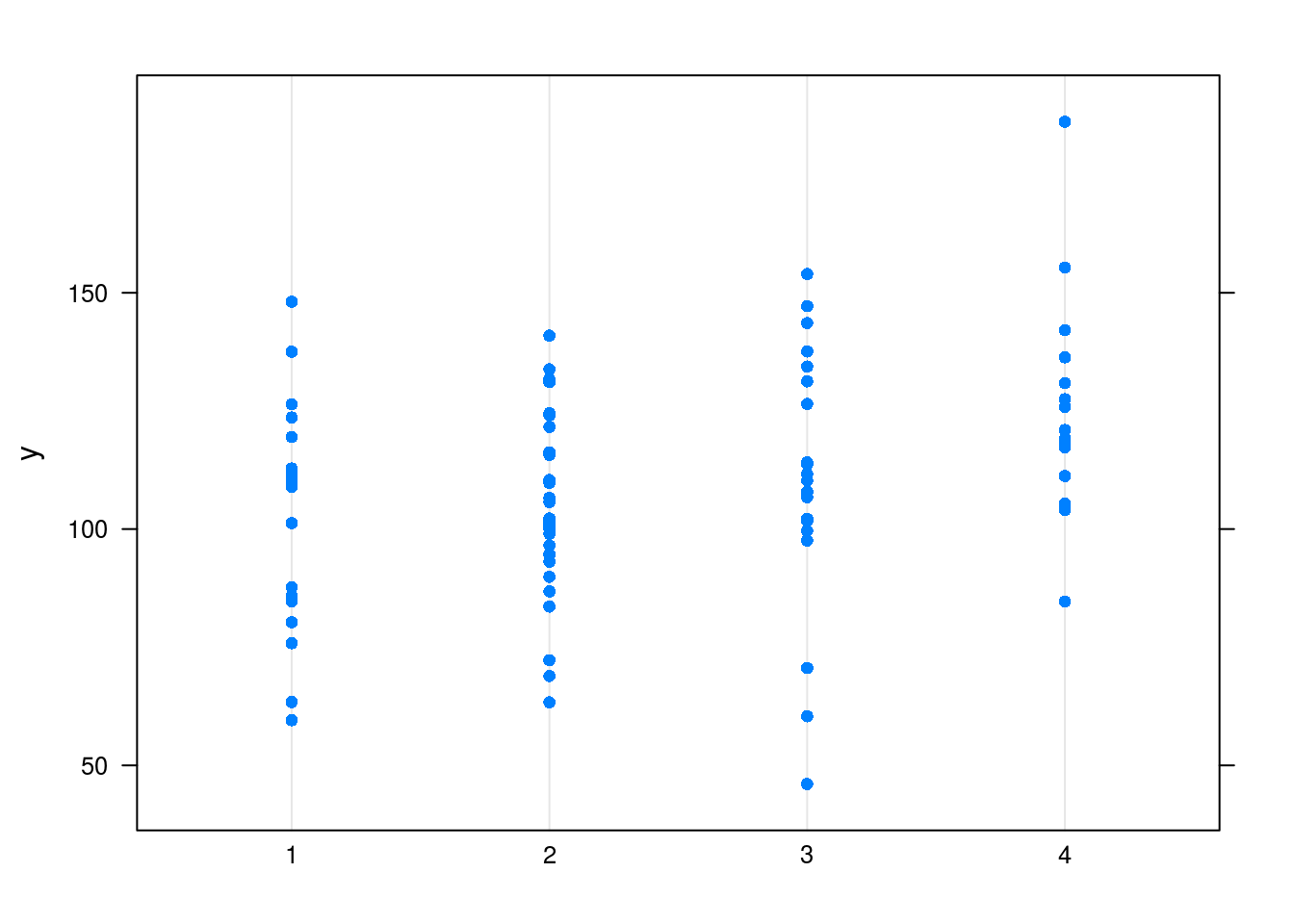``with(dat1, boxplot(y ~ group))``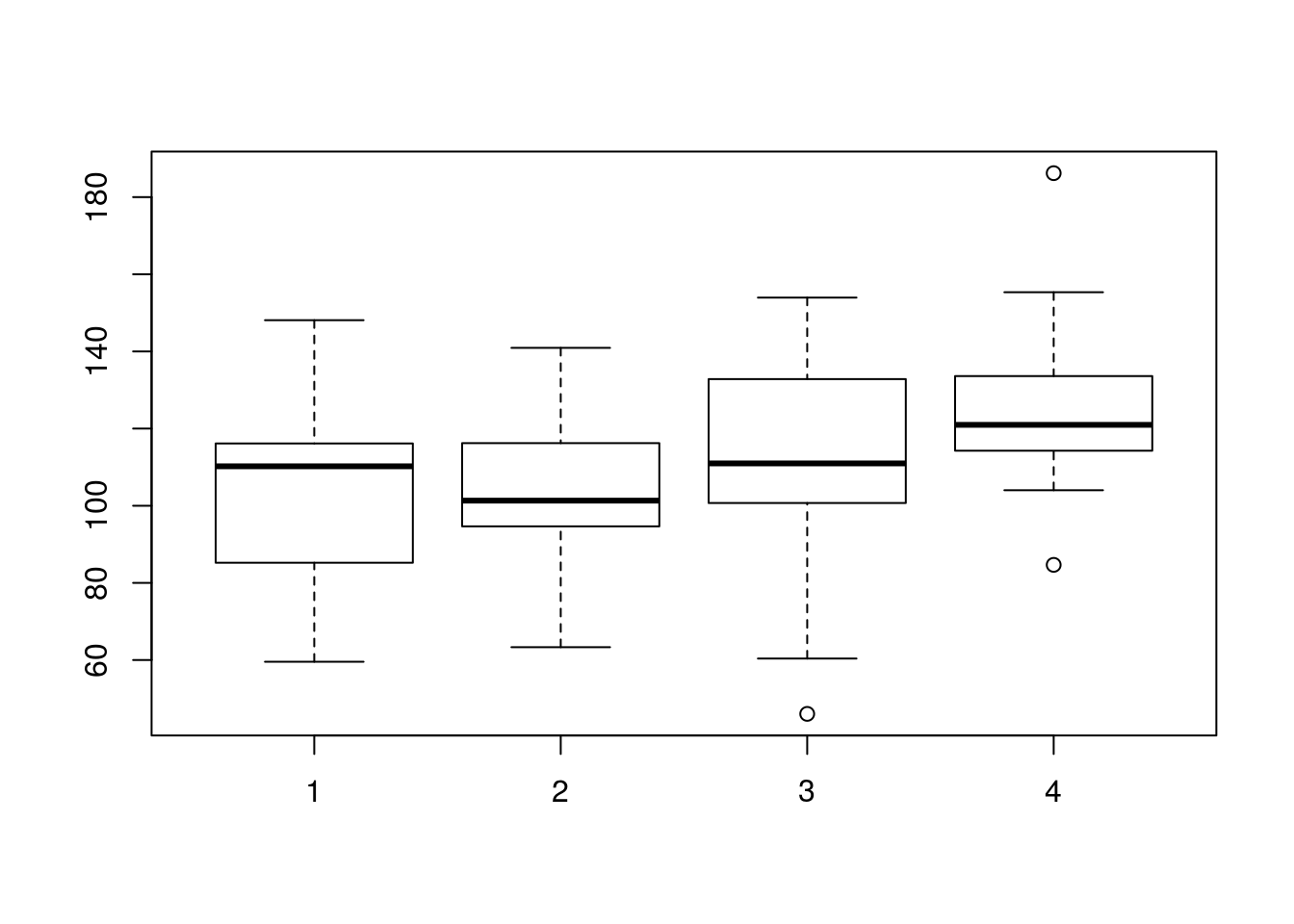``````require(ggplot2)

ggplot(dat1, aes(group, y)) +
geom_violin() + geom_dotplot(binaxis='y', stackdir='center', dotsize=.5)``````
``## `stat_bindot()` using `bins = 30`. Pick better value with `binwidth`.``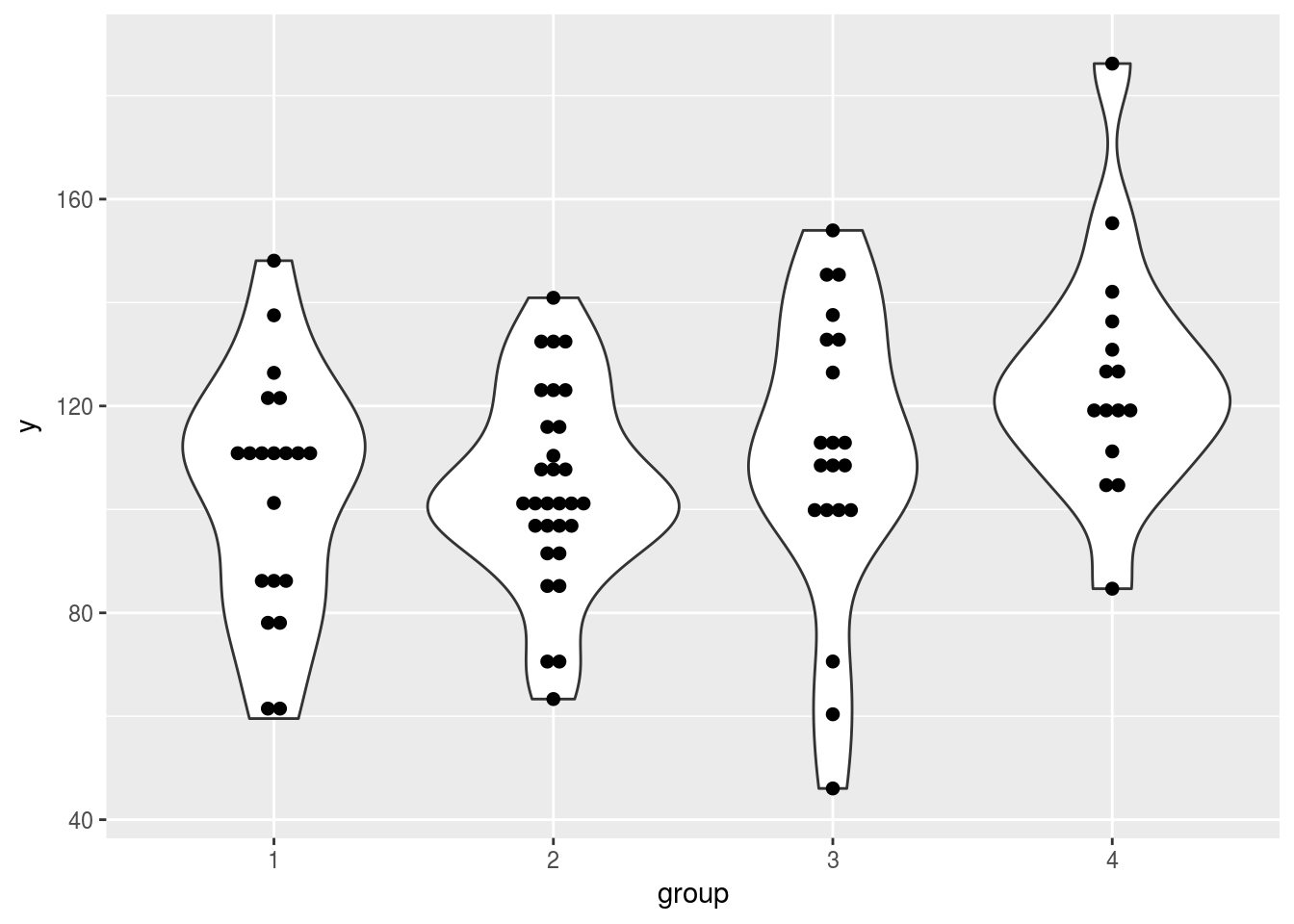``````require(ez)

ezPlot(dat1, y, wid=subj, between=group, x=.(group))``````
``````## Warning: Data is unbalanced (unequal N per group). Make sure you specified
## a well-considered value for the type argument to ezANOVA().``````
``````## Warning in ezStats(data = data, dv = dv, wid = wid, within = within,
## within_full = within_full, : Unbalanced groups. Mean N will be used in
## computation of FLSD``````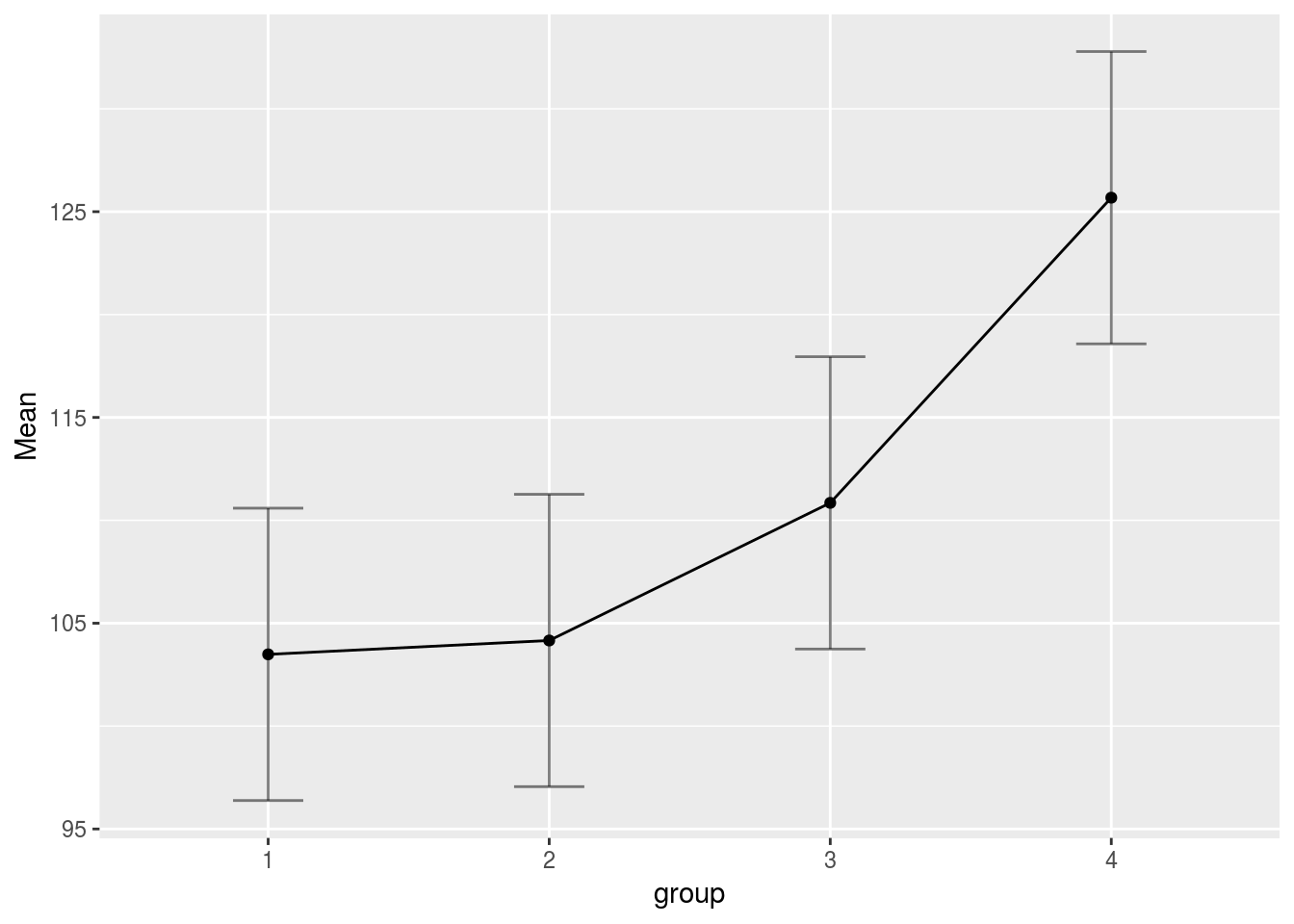## Anova

``ezANOVA(dat1, dv=y, wid=subj, between=group)``
``````## Warning: Data is unbalanced (unequal N per group). Make sure you specified
## a well-considered value for the type argument to ezANOVA().``````
``````## \$ANOVA
##   Effect DFn DFd        F          p p<.05       ges
## 1  group   3  81 3.409065 0.02139588     * 0.1121069
##
## \$`Levene's Test for Homogeneity of Variance`
##   DFn DFd      SSn      SSd         F         p p<.05
## 1   3  81 499.0771 20586.27 0.6545666 0.5823939``````

To conduct post-hoc tests comparing all pairs of means:

``````hsd <- TukeyHSD(aov(y ~ group, data=dat1))
print(hsd)``````
``````##   Tukey multiple comparisons of means
##     95% family-wise confidence level
##
## Fit: aov(formula = y ~ group, data = dat1)
##
## \$group
##           diff        lwr      upr     p adj
## 2-1  0.6734241 -16.952321 18.29917 0.9996365
## 3-1  7.3623076 -11.945729 26.67034 0.7496736
## 4-1 22.1939720   1.338909 43.04903 0.0324969
## 3-2  6.6888835 -10.936861 24.31463 0.7523986
## 4-2 21.5205479   2.212512 40.82858 0.0227903
## 4-3 14.8316644  -6.023398 35.68673 0.2510137``````
``plot(hsd)``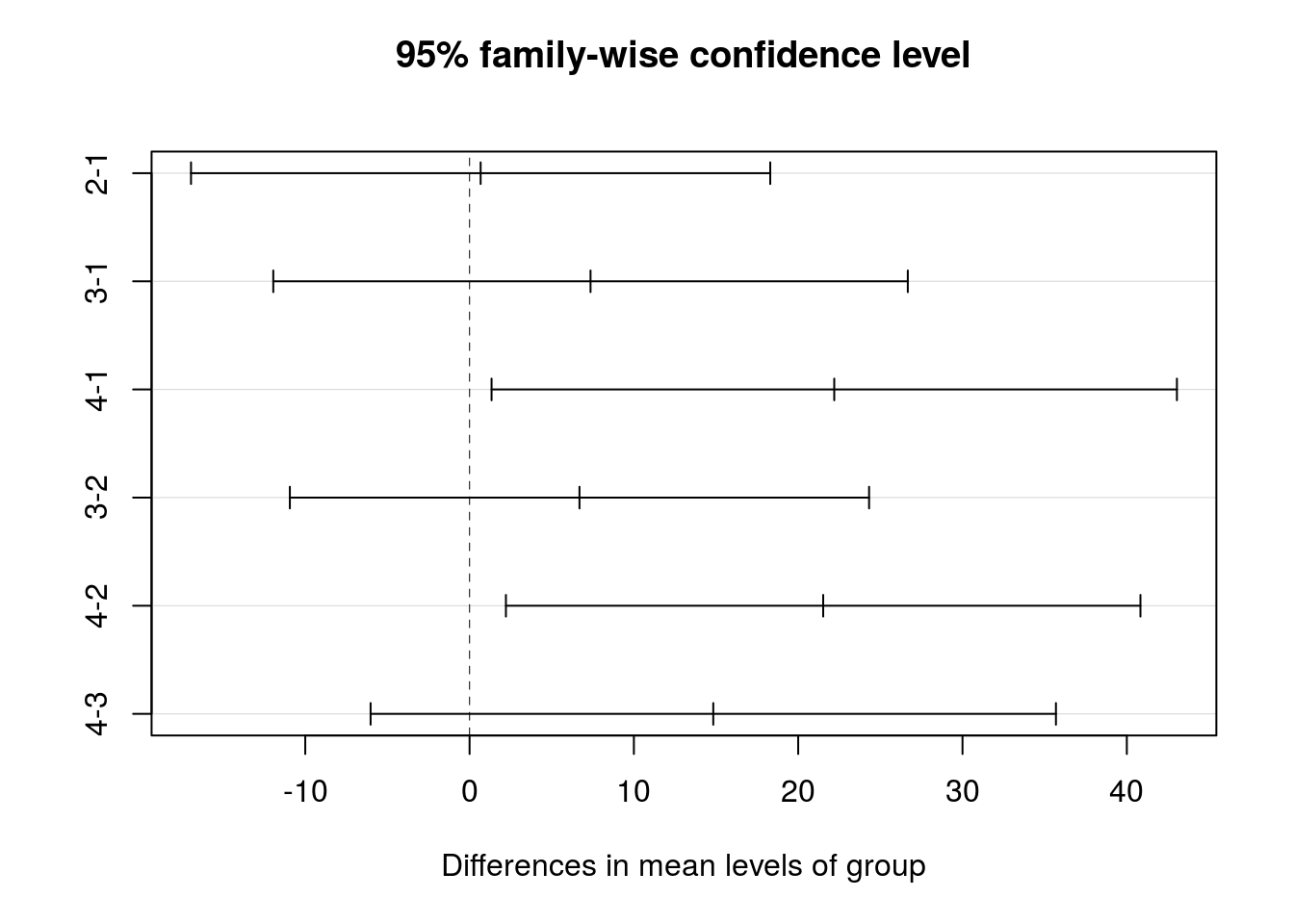If you worry about heteroscedasticity, you can check the diagnostic plots of `lm`:

``````par(mfcol=c(2, 2))
plot(lm(y ~ group, data=dat1))``````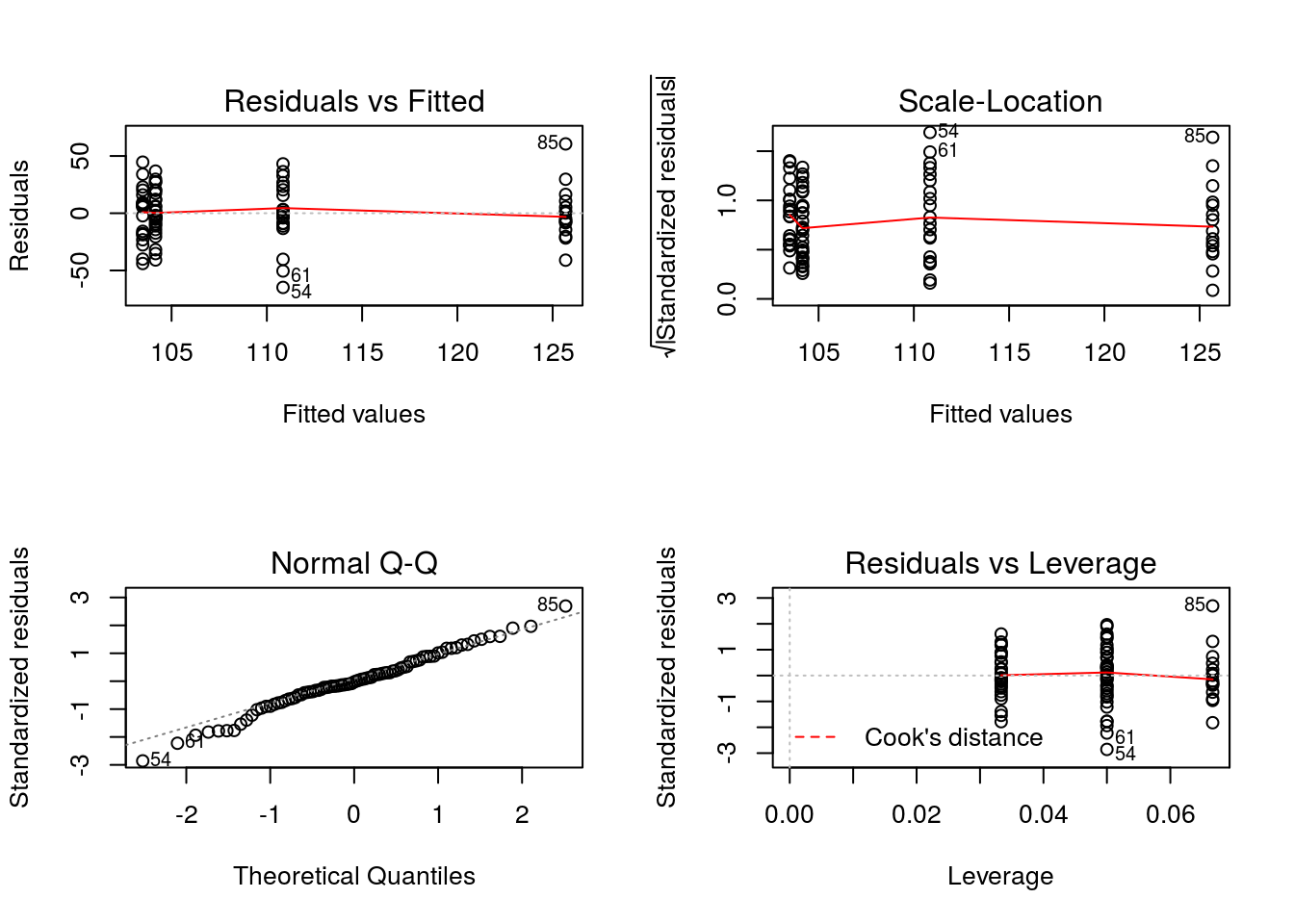If heteroscedasticity is severe, it will be visible on the plots of the “residuals vs. fitted” plot.

# S*T Design (one-way Anova within subjects)

In this design, the factor ‘Subject’ is crossed with a factor ‘Treatment’, that is, there is one data point per subject and treatment.

``````ns <- 20
means <- c(100, 110, 100, 130)
nt <- length(means)
sd <- 20

dat2 <- data.frame(
subj=gl(ns, nt),
treat=gl(nt, 1, nt*ns))

dat2\$y <- rep(means, ns) + rep(rnorm(ns, sd=20), each=nt) + rnorm(nt*ns, sd=20)

str(dat2)``````
``````## 'data.frame':    80 obs. of  3 variables:
##  \$ subj : Factor w/ 20 levels "1","2","3","4",..: 1 1 1 1 2 2 2 2 3 3 ...
##  \$ treat: Factor w/ 4 levels "1","2","3","4": 1 2 3 4 1 2 3 4 1 2 ...
##  \$ y    : num  105.6 103.3 69.1 123 69.6 ...``````
``head(dat2)``
``````##   subj treat         y
## 1    1     1 105.59941
## 2    1     2 103.30880
## 3    1     3  69.06811
## 4    1     4 123.01079
## 5    2     1  69.58211
## 6    2     2  97.29196``````

We first plot the raw data, per subject:

``````require(lattice)
xyplot(y ~ treat | subj, data=dat2, type='b')``````